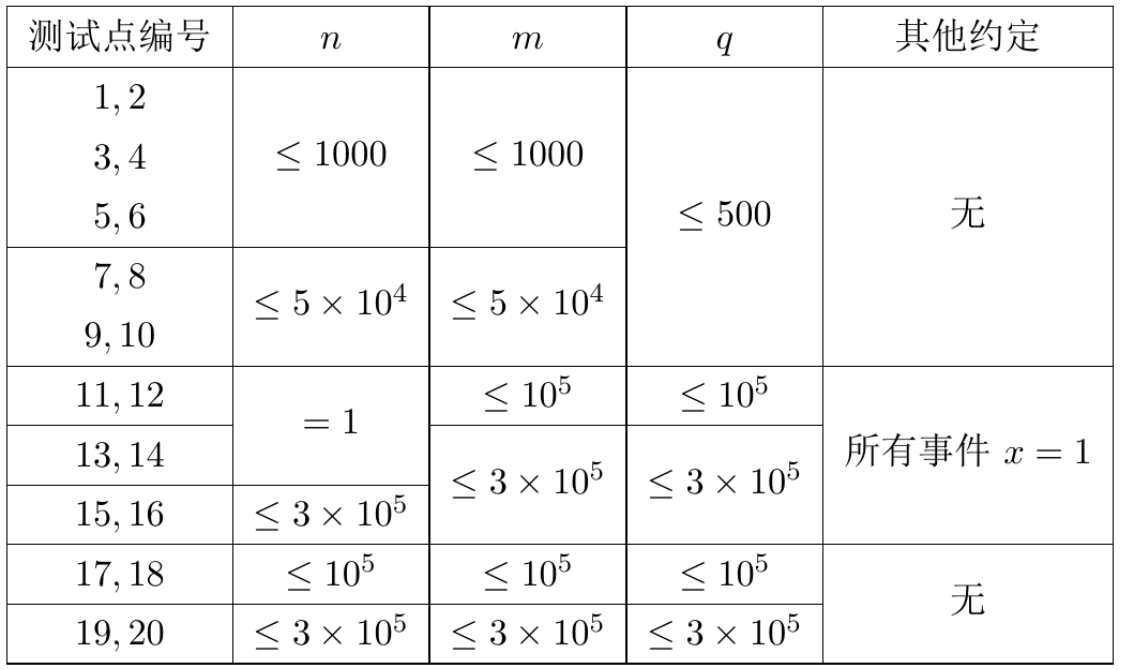# 洛谷 P3960 NOIp2017提高组 列队 题解【splay】【模拟】

领教了splay的强大/考场上只拿了无脑30分

## 【问题描述】

Sylvia 是一个热爱学习的女孩子。

Sylvia参加了学校的军训。众所周知，军训的时候需要站方阵。 Sylvia所在的方阵中有$n\times m$名学生，方阵的行数为$n$，列数为$m$

1到$n\times m$编上了号码（参见后面的样例）。即：初始时，第$i$行第$j$列的学生的编号是$(i-1)\times m+j$

1. 向左看齐。这时第一列保持不动，所有学生向左填补空缺。不难发现在这条指令之后，空位在第$x$行第$m$列。

2. 向前看齐。这时第一行保持不动，所有学生向前填补空缺。不难发现在这条指令之后，空位在第$n$行第$m$列。

## 【输入格式】

1行包含3个用空格分隔的正整数$n,m,q$，表示方阵大小是$n$行$m$列，一共发生了$q$次事件。

## 【输入输出样例 1】

2 2 3
1 1
2 2
1 2

1
1
4

## 【输入输出样例 1 说明】

$\begin{bmatrix} 1&2\\ 3&4\end{bmatrix} \Rightarrow \begin{bmatrix} &2\\ 3&4\end{bmatrix}\Rightarrow \begin{bmatrix}2&\\ 3&4\end{bmatrix}\Rightarrow \begin{bmatrix}2&4\\3&\end{bmatrix} \Rightarrow \begin{bmatrix}2&4\\3&1\end{bmatrix}$

$\begin{bmatrix}2&4\\3&1\end{bmatrix}\Rightarrow \begin{bmatrix}2&4\\3& \end{bmatrix}\Rightarrow \begin{bmatrix}2&4\\3& \end{bmatrix}\Rightarrow \begin{bmatrix}2&4\\3& \end{bmatrix}\Rightarrow \begin{bmatrix}2&4\\3&1\end{bmatrix}$

$\begin{bmatrix}2&4\\3&1\end{bmatrix}\Rightarrow \begin{bmatrix}2& \\3&1\end{bmatrix}\Rightarrow \begin{bmatrix}2& \\3&1\end{bmatrix}\Rightarrow \begin{bmatrix}2&1\\3& \end{bmatrix}\Rightarrow \begin{bmatrix}2&1\\3&4\end{bmatrix}$

1的同学离队，这时空位在第一行第一列。接着所有同学向左标齐，这时编号为2的同学向左移动一步，空位移动到第一行第二列。然后所有同学向上标齐，这时编号为4的同学向上一步，这时空位移动到第二行第二列。最后编号为1的同学返回填补到空位中。

【数据规模与约定】## 题解：

首先看数据范围，可以看出，前30分用$O(nq)$的模拟是可以过的，前50分都是可以离散化行数做的，11~16这些点维护一个简单操作的平衡树就可以了。其实这道题给的可做分还是很多的。

看到全部数据的范围是300,000就要考虑$O(q\log n)$的做法，于是可以用splay来做。（反正考场上是怎么也想不到的）

而每次队列的变动只会在每一行，最后一列上，因此我们可以维护$n+1$棵splay，它不具有BST的性质，只是按顺序维护了$m$个节点。每次出队时把第$x$棵splay的第$y$个节点删掉，放到最后一列的splay的最后一行去，同时取出并删掉最后一列的第$x$个元素，插入第$x$行的那棵splay的最后。这样每次操作只需要平衡树的复杂度，也就是$O(q\log (n+m))$。不过300,000棵有300,000个节点的平衡树，空间说什么也爆了。

因为我们要维护的是300,000×300,000个点，而操作只有300,000次，并且每次操作一定有很多点没有出过队列。如果我们访问$(i,j)$位置上的数字，那从$(i,j+1)$到$(i,m)$位置上的数都要整体左移。在300,000次的操作中，这样的区间也不会超过600,000个，因此我们可以用splay维护区间，当访问到区间内的一个数$x$时，就把区间所在的节点$[l,r]$分裂为3个，分别代表$[l,x),{x},(x,r]$，把原来的节点改为$[l,x)$，在它的右孩子插入$(x,r]$，把它原来的右孩子放在右孩子的右孩子处，就维护了splay顺序的性质。

不过在这个过程中要注意一点，就是当被分裂的点的区间长度为1时，直接拿它的左子树中最右边一个或右子树中最左边一个顶掉自己的位置，当被分裂的点没有孩子时返回NULL即可。

因为我们还额外维护了最后一列，所以每一行只用存$m-1$个数，最后一列是会变的。当某一行的区间长度和小于$m-1$时，就去最后一列找这一行最后一列的元素插入，接着调整最后一列。

在这个题中，插入总是在某行或某列的最后，因此Insert写的十分简单。而注意分裂时根据splay的性质把要分裂的点旋到根上会更好地保持树的平衡（不管怎样以后splay做什么操作都得把它旋到根上…）。并且这个题中几乎所有的查找都是根据区间第几位来查找的，区间改变时注意改变区间大小。

于是一个比正常splay代码还要短一截的神奇分裂splay就能过掉这个题了。。。

## Code：

#include<cstdio>
#include<cstring>
struct node
{
long long l,r;
int sz;
node *s;
node(long long l,long long r)
{
this->l=l;
this->r=r;
sz=r-l+1;
s=s=NULL;
}
node()
{
s=s=NULL;
}
int getw()
{
if(!this)
return 0;
return sz;
}
int getd(int x)
{
if(x>(s->getw())&&x<=(s->getw()+r-l+1))
return -1;
return x>s->getw();
}
void maintain()
{
sz=s->getw()+s->getw()+r-l+1;
}
}*root;
void Insert(node *&rt,long long x)//在rt的最右边插入x
{
if(!rt)
{
rt=new node(x,x);
return;
}
Insert(rt->s,x);
rt->maintain();
}
void Rotate(node *&rt,int d)
{
node *tmp=rt->s[d];
rt->s[d]=tmp->s[d^1];
tmp->s[d^1]=rt;
rt->maintain();
rt=tmp;
rt->maintain();
return;
}
void splay(node *&rt,int x)
{
int d1=rt->getd(x);
if(d1==-1)
return;
int d2=rt->s[d1]->getd(x-(d1==1)*(rt->s->getw()+rt->r-rt->l+1));//注意减掉左子树和自己
if(d2==-1)
{
Rotate(rt,d1);
return;
}
splay(rt->s[d1]->s[d2],x-(d1==1)*(rt->s->getw()+rt->r-rt->l+1)-(d2==1)*(rt->s[d1]->s->getw()+rt->s[d1]->r-rt->s[d1]->l+1));
if(d1==d2)
{
Rotate(rt,d1);
Rotate(rt,d1);
}
else
{
Rotate(rt->s[d1],d2);
Rotate(rt,d1);
}
return;
}
long long ans;
void Split(int num,node *&rt,long long x,int flag)//分出第x个,flag表示是否打印答案
{
splay(rt,x);
x-=rt->s->getw();
ans=rt->l+x-1;
if(flag)
printf("%lld\n",ans);
if(rt->l==rt->r)
{
if(rt->s)
{
splay(rt->s,rt->s->getw());
rt->s->s=rt->s;
rt->s->maintain();
rt=rt->s;
rt->maintain();
}
else if(rt->s)
{
splay(rt->s,1);
rt->s->s=rt->s;
rt->s->maintain();
rt=rt->s;
rt->maintain();
}
else
rt=NULL;
return;
}
node *tmp=rt->s;
long long l=rt->l,r=rt->r;
rt->r=l+x-2;
if(l+x>r)
{
rt->maintain();
return;
}
else if(rt->l>rt->r)
{
rt->l=l+x;
rt->r=r;
rt->maintain();
return;
}
rt->s=new node(l+x,r);
rt->s->s=tmp;
rt->s->maintain();
rt->maintain();
Rotate(rt,1);
return;
}
int main()
{
long long n,m,q;
scanf("%lld%lld%lld",&n,&m,&q);
for(long long i=1;i<=n;i++)
root[i]=new node((i-1)*m+1,i*m-1);
root=new node();
node *right=new node();
root=new node(m,m);
root->sz=n;
right=root;
for(long long i=2;i<=n;i++)
{
right->s=new node(i*m,i*m);
right->s->sz=n-i+1;
right=right->s;
}
splay(root,n);
long long x,y;
for(int i=1;i<=q;i++)
{
scanf("%lld%lld",&x,&y);
if(y<m)
{
Split(x,root[x],y,1);
Insert(root,ans);
splay(root,n);
Split(0,root,x,0);
Insert(root[x],ans);
splay(root[x],m-1);
}
else
{
Split(0,root,x,1);
Insert(root,ans);
splay(root,n);
}
}
return 0;
}

### 2 说点什么0 Followers

Most reacted comment
0 Comment authors
Recent comment authors
SubscribewpDiscuz